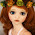## Random Forest

Random Forest is a machine learning technique to automatically classify data into their most likely category based on a training sample. For example, you may want to categorize a movie as good or bad, a drug as effective or ineffective on a given patient, a bitmap image into the digits 0-9.

To perform this feat, before RF 'looks' at a sample with known categories, and 'learns' from it. This is called the 'training' process.

Since it uses a training data to learn, the method is called supervised learning, as opposed to unsupervised learning where a data is categorized into its most likely clusters without having any hint of what those clusters may be from any previously available data sources.

Some other supervised learning techniques are Logistic Regression, Neural Networks, Support Vector Machines, and Gradient Boosting Machines.

A Random Forest, as the name indicates, generates a collection of trees - viz. Decision Trees - at the end of the learning process. Each tree by itself is a predictor. To categorize a new observation, it is run through all the trees, and the results are aggregated.

## Testing Random Forest

How well does it work?

To test it, I created a data that where I color a point $$(x,y)$$ as Green if $$y>\sin(x)$$, otherwise I call it Red.

Note conventional methods like Logistic Regression, $$P(green)=\left({1+e^{-(\alpha+\beta_1 x + \beta_2 y)}}\right)^{-1}$$, would fail on this data, as it depends on the relationships being linear.Test Function

Then I created samples of different sizes consisting of $$(x,y)$$ points, applied the red/green labeling for training the RF.Training Samples

As I was interested to see the quality of the prediction varies on the amount of data, I fed the above samples separately. The number of trees that RF generates, which upto a point increases the predictive power, was also varied. It was RF's job to come up with the function just by looking these points.Guess made by Random Forest

Looking at this pictures, it it apparent that RF recreated the functions very accurately, even with as low as 100 observations - for which it may actually be difficult for a person to guess the function.

For our simple data, the incremental gain by increasing the number of trees from 50 to 1000 is small.

## Confidence

RF has a natural candidate that can be used to estimate how confident it is for each prediction. If there are $$n$$ trees, we can simply compute the variance of the predictions $$v_k=\frac{1}{n}\sum_{i=1}^n(\hat p_k-\frac{1}{n}\sum(\hat p_k))$$ for each observation.

A sense of the confidence can be seen by looking at the white region in the charts above. Below is a plot of the relative standard deviation which brings it out clearly.Relative Uncertainty of Random Forest

Darker areas correspond to regions of higher uncertainty.

Observe that the most uncertain regions are at the boundary region, as one would expect, since it is at the boundary you will be most unsure about what color a point will have.

Also as the size of the training sample is increased, uncertainty recedes more and more towards the boundary of the classes.

## Conclusion

Per each data point, the variance of outcomes by all the trees can be used to estimate the confidence in its prediction. It is valuable especially for estimating continuous attributes (though the simple example above demonstrates this on discrete outcomes), but it is generally overlooked. In a scenario where a bad decision is costly, the confidence can be used to filter the data before taking a decision.

1.would you mind sharing your R code?

2.Thanks this is a great visual example of confidence. I particularly like how the areas with few data points have very low confidence. It would be interesting to see the visualisations for even fewer trees, e.g. 5 or 10.

3.great blog with useful information..
4.